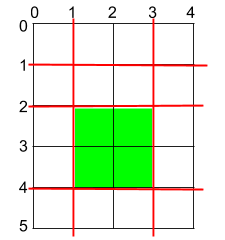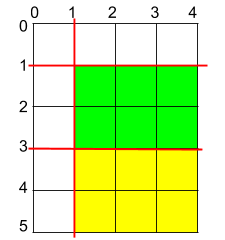# 切割后面积最大的蛋糕

## 1465. 切割后面积最大的蛋糕 (Medium)```输入：h = 5, w = 4, horizontalCuts = [1,2,4], verticalCuts = [1,3]

``````输入：h = 5, w = 4, horizontalCuts = [3,1], verticalCuts = 

```输入：h = 5, w = 4, horizontalCuts = , verticalCuts = 

```

• `2 <= h, w <= 10^9`
• `1 <= horizontalCuts.length < min(h, 10^5)`
• `1 <= verticalCuts.length < min(w, 10^5)`
• `1 <= horizontalCuts[i] < h`
• `1 <= verticalCuts[i] < w`
• 题目数据保证 `horizontalCuts` 中的所有元素各不相同
• 题目数据保证 `verticalCuts` 中的所有元素各不相同

[贪心] [数组] [排序]